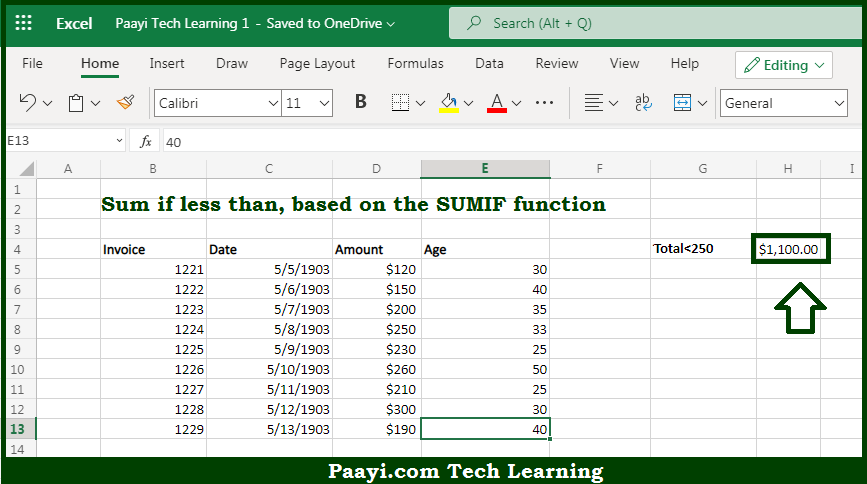# Learn How to SUM If Less Than in Microsoft Excel

Written by | 0 Comments | 648 Views

In this article, you will learn how to SUM various things in Microsoft Excel using a single/combination(s) of functions. You will also know how to SUM If Less Than and see the generic formula.

SUM If Less Than in Microsoft Excel

The main purpose of this formula is to sum if less than. Here we will learn how to sum if one cell is grate than another in the workbook in Microsoft Excel. That implies, with the help of a formula based on the SUMIF function you can able to sum if less than. So, with the help of this formula, you can able to sum if one cell is grate than another in the workbook in Microsoft Excel.

General Formula to SUM If Less Than

=SUMIF(range,"<num")

The Explanation for the SUM If Less ThanSo we know that with the help of the given formula above you can able to sum if less than. Here we will learn how to sum if one cell is grate than another in the workbook in Microsoft Excel. As we know that the SUMIF function supports  logical operators efficiently, which are -  "=",">",">=", etc. In the example provided here we want to match amounts less than the given value, and the "criteria range" is the same as the "sum range". That is why there is no need to enter the sum range as a final argument. So, with the help of this formula, you can able to sum if one cell is grate than another in the workbook in Microsoft Excel.